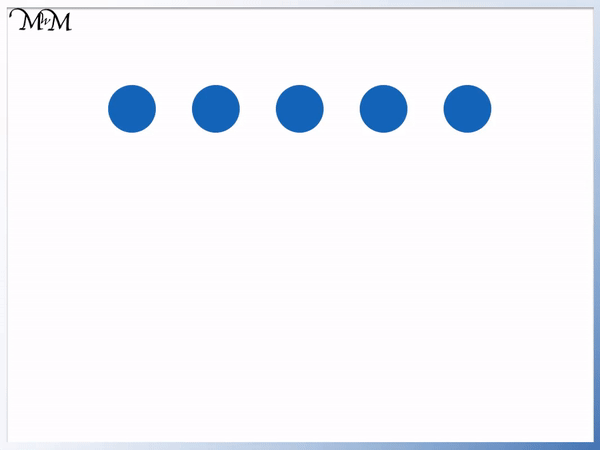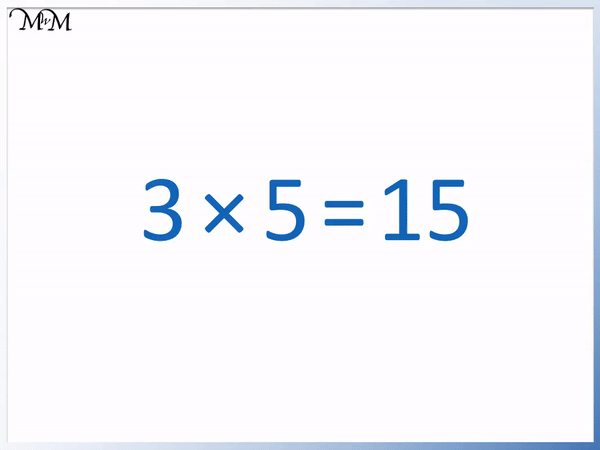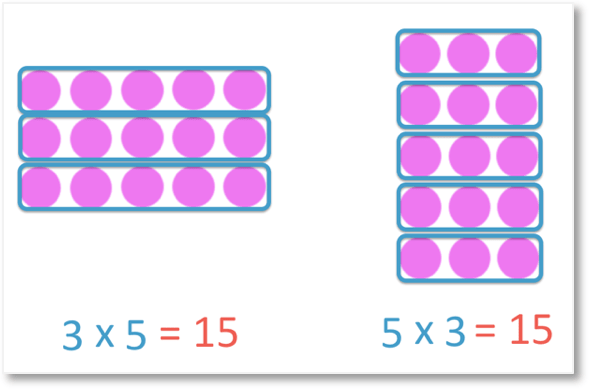# Multiplication Sentences

Multiplication Sentences• An array is a rectangular collect of objects which represent a multiplication.
• We have 3 rows of 5 counters.
• We have 3 equal groups of 5 or 3 lots of 5.
• The multiplication sign, ×, means lots of.
• 3 lots of 5 can be written as 3 × 5.
• A multiplication sentence is made up of 3 numbers, a multiplication sign, × and an equals sign, =.
• The number before the × sign tells us how many groups we have.
• The number after the × sign tells us how many are in each group.
• The number at the end, after the equals sign is the total number of items.
• There are 15 counters in total so we write 3 × 5 = 15.

The larger number comes after the equals sign and tells us how much we have in total.

The number before the multiplication sign is how many groups we have and the number after the sign is how many in each group.• We will write a multiplication sentence to describe how many wings we have in total.
• There are 3 butterflies, so we write 3 in front of the multiplication sign.
• There are 2 wings on each butterfly and so, the 2 goes after the multiplication sign.
• There are 6 wings in total on the 3 butterflies so we write 6 after the equals sign.
• The multiplication sentence is 3 × 2 = 6.
• We can read this as 3 lots of 2 is 6.# Multiplication Sentences

## What is a Multiplication Sentence?

A multiplication sentence is made up of 3 numbers. The first number, before the multiplication sign tells us how many equal groups we have. The second number, after the multiplication sign tells us how many are in each group. The 3rd number comes after the equals sign and is how many there are in total.

An example of a multiplication sentence is 3 × 5 = 15. The multiplication sentence is made up of 3 numbers. 2 next to the multiplication sign and one at the end, after the equals sign.This multiplication sentence means 3 lots of 5 makes a total of 15.

It is helpful to remember that when writing multiplication sentences for whole numbers, the largest number goes at the end, after the equals sign.

A multiplication sentence is a mathematical way to write the total number of objects that are in equal groups.

For example, below we have 3 butterflies.

Each butterfly has 2 wings.

To find the total number of wings we add the 2 wings on the first butterfly to the 2 wings on the second butterfly to the 2 wings on the third butterfly.

If we add 2 + 2 + 2 we get 6. There are 6 wings in total.It is easier to think of this as 3 groups of 2 wings. Each butterfly has 2 wings and there are 3 butterflies.

We can say that we have 3 lots of 2, which is 6 in total.

The multiplication sign is ×. The multiplication sign is used instead of writing ‘lots of’ or ‘equal groups of’.

SO we can write 3 × 2 = 6, which means that 3 lots of 2 is equal to 6. It is quicker, easier and takes up less space to write compared to writing the multiplication sentence in words.

## Writing Multiplication Sentences for Arrays

There are 2 multiplication sentences that can be written for an array. To write a multiplication sentence for an array use the following steps:

1. Count the number of rows and write this number.
2. Write the multiplication sign, ×.
3. Count the number of columns and write this number after the multiplication sign.
4. Write an equals sign, =.
5. Count the total number of objects in the array and write this number after the equals sign.
6. To find the other multiplication sentence, switch the two numbers either side of the multiplication sign.

Arrays are often used to teach multiplication. An array is a rectangular collection of objects (usually counters) arranged in equal rows and columns.

Here is an example of a multiplication array.

We have 5 counters in each row.We have 3 rows of 5 in the array. Every row contains 5 counters.

We can say that we have 3 rows of 5. Because every row in the array has the same number of counters, we can say that we have 3 ‘equal groups of’ 5 or simply, 3 ‘lots of’ 5.

When writing a multiplication sentence for this array, we can replace the words ‘lots of’ with a multiplication sign, ×.

We can write 3 × 5 to mean 3 equal lots of 5.

The number after the equals sign tells us how many counters we have in total in the array. If we count all of the counters, we can see that we have 15.

The multiplication sentence for this array is written as 3 × 5 = 15.This multiplication sentence means 3 lots of 5 makes a total of 15.

When writing a multiplication sentence with whole numbers, the two smaller numbers come first and go next to the multiplication sign. The larger number comes last, after the equals sign.

In a multiplication sentence, the two numbers either side of the multiplication sign are called factors and the number after the equals sign is called the multiple.

If you wrote the two smaller numbers the other way around as 5 × 3 = 15 instead of 3 × 5 =15, this does not matter when talking about this array.

It would mean 5 lots of 3. We can still think of this array as representing 5 lots of 3 because we have 5 columns, each containing 3 counters.

We have 3 rows containing 5 counters or 5 columns each containing 3 counters.

So for this array, the multiplication sentences are:

3 × 5 = 15 or

5 × 3 = 15.

The total must come after the equals sign.

Here is another example of writing a multiplication sentence for an array.

In this array we have 4 counters in each column.

We have 6 columns.

There are 6 columns of 4. Because there are an equal number of counters in each column, we can write this as a multiplication.We have 6 lots of 4. Remember that we can replace the words lots of with a multiplication sign.

We can write the multiplication sentence for this array as 6 × 4 = 24.

There are 24 counters in total. We can count the counters individually our repeatedly add 4 + 4 + 4 + 4 + 4 + 4 to get 24.

6 × 4 = 24 means that 6 lots of 4 makes a total of 24.

The first number tells us how many groups we have. The second number tells us how many are in each group and the final number tells us how many there are in total.The two numbers being multiplied are called factors and the number at the end after the equals sign is called the multiple.

Factors multiply together to make a multiple.

Remember that when writing a multiplication sentence for an array, there are two possible options.

We have 6 columns of 4 or 4 rows of 6.

We can switch the two numbers either side of the multiplication sign.

We have 6 × 4 = 24 or

4 × 6 = 24.

## How to write Multiplication Sentences

To write a multiplication sentence use the following steps:

1. Count the number of equal groups and write this number down.
2. Write a multiplication sign.
3. Count the number of objects in each group and write this number after the multiplication sign.
4. Write an equals sign.
5. Count the total number of objects and write this number after the equals sign.

Here is an example of writing a multiplication sentence to describe a problem.

We have 2 ladybirds and there are 6 legs on each ladybird.

In total there are 12 legs.

We are asked to write a multiplication sentence to show how many legs there are in total.The first step is count the number of equal groups. The legs are in two groups. Each ladybird is a group of legs.

We have 2 groups, so we write 2.

There are 6 legs in each ladybird, so 6 legs in each group.

2 lots of 6 legs can be written as 2 × 6.

If we count the total number of legs, we can see that there are 12.

This total number goes after the equals sign.

The multiplication sentence to describe this problem is 2 × 6 = 12. It means 2 lots of 6 legs is 12 legs.

Here is another example of writing a multiplication sentence for a problem.

We have 5 bunches of flowers and in each bunch there are 4 flowers.

If we count the individual flowers, there are 20 in total.We can say that we have 5 groups of 4 flowers.

We can write this as 5 × 4.

The total number of flowers goes at the end, after the equals sign.

The multiplication sentence is 5 × 4 = 20.Now try our lesson on Order of Multiplication where we learn that it does not matter in which order we multiply two numbers.